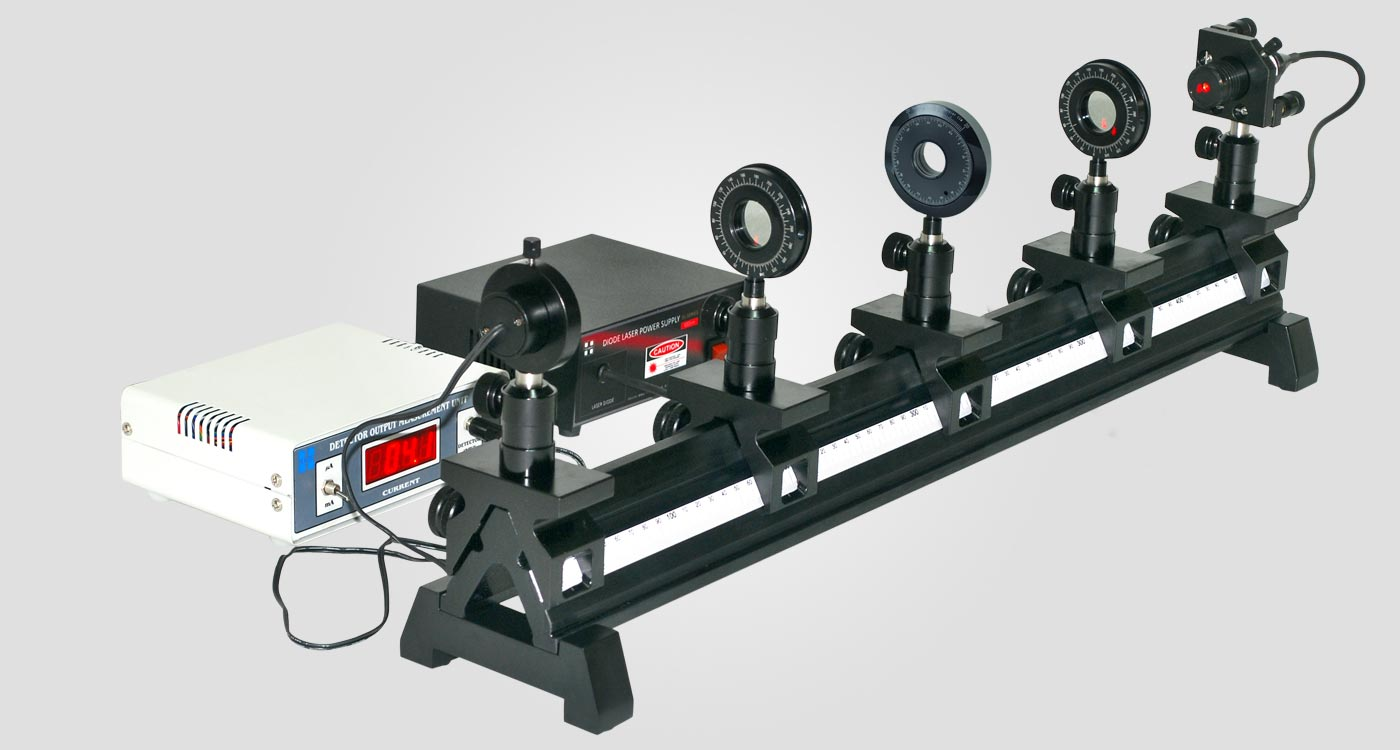Apparatus for the Study of

Polarisation by Waveplates

Model: HO-ED-P-07

A wave plate or retarder is an optical device that alters the polarization state of a light beam travelling through it. A typical wave plate is simply a birefringent crystal like quartz ,calcite etc. with a particular thickness.

If a beam of parallel light passes perpendicularly through a wave plate, the light beam is split into two components due to its double refracting properties. The two components have planes of oscillation perpendicular to each other and slightly different phase velocities. For a quarter-wave plate, the thickness of the quartz plate is chosen in such a manner that the light component whose electric field vector oscillates parallel to the rotation lever lags by a λ/4 behind other perpendicular oscillating light component. For a half-wave plate the thickness is chosen so that the created phase difference is λ/2.

Experiment Examples

Intensity measurement as a function of analyzer angle

The refractive index of the solid prism using the formula,

n    =    sin  ( (α+β) / 2 )   /   sin (α/2)

Where α is angle of the prism & β is angle of minimum deviation.Polarization study by quarter wave plate - elliptical and circular polarization.

Dispersive power,

ω = (n2- n1) / (n - 1)

where,

n1 and n2 are the refractive indices of the prism for any two colours, and n = (n1 + n2 ) / 2

Polarization study by half wave plate - change of polarization axis.

Replace the solid prism with hollow prism containing the liquid for which the refractive index to be calculated.
The experimental procedure is same as solid prism.

Verification of Malus' law

If a parallel beam of monochromatic light is incident normally on the face of a plane transmission diffraction grating, bright diffraction maxima are observed on the other side of the grating. These diffraction maxima satisfy the grating condition:

N sin qn = nl

Where N is the the number of rulings in grating,

qn is the angle of diffraction of the nth maximum.

n is the order of spectrum which can take values 0, ±1, ±2, ±3 …….

l is the wavelength of the incident light.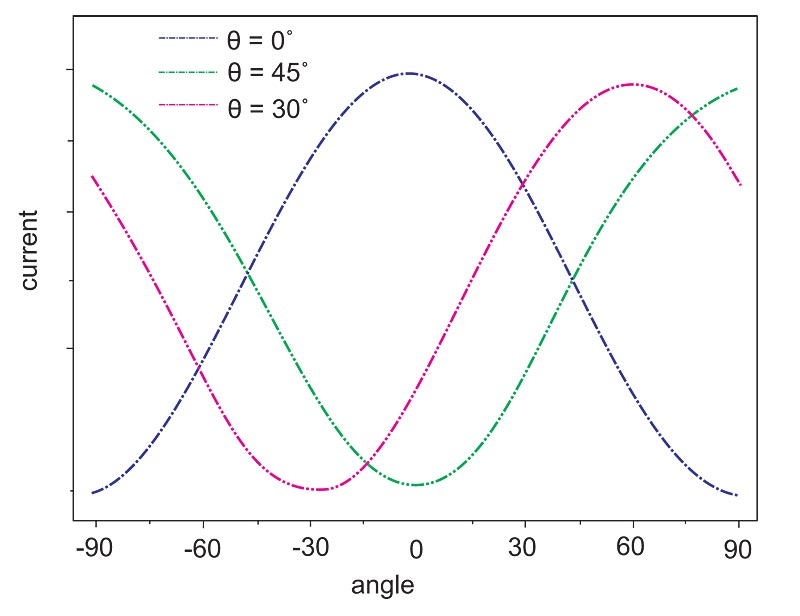Fig: Current as a function of the analyzer position for various half wave plate positions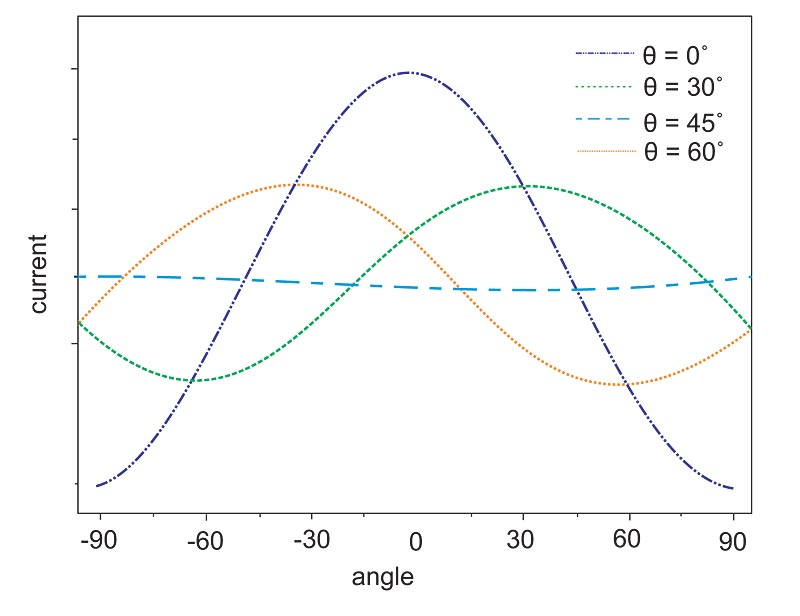Fig: Current as a function of the analyzer position for various quarter wave plate positions

In this experiment a laser beam passes through a polarizer, quarter-wave or half-wave plate, analyzer and finally falls on the detector. The polarization of the emergent light is investigated at different angles between the optic axis of the wave plates and the direction of the incident light. The detector output current is recorded at different analyzer angles. The change of polarization state and axis can be studied from the graph between detector output current and analyzer angle.

Scope of Supply

Polarizer with mount

Model No: ED-P-07-PRM
Material
:
Sheet Polarizer
Rotation
:
360 degree
Quantity
:
1 no.
Quantity
:
1 no.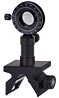Analyzer with mount

Model No: ED-P-07-ARM
Material
:
Sheet Polarizer
Rotation
:
360 degree
Quantity
:
1 no.
Quantity
:
1 no.Half wave & quarter wave plates with mount

Model No: ED-P-07-WPM
Material
:
Quartz Crystal
Diameter Tolerance
:
± 0.2 mm
Surface Quality
:
20 - 10 (Scratch-dig)
Surface Flatness
:
λ / 4 @ 632.8nm
AR Coating
:
Multilayer
Retardance Accuracy
:
< λ / 300
Quantity
:
1 no. each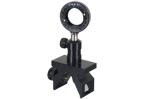Diode laser with power supply

Model No: ED-P-07-DLPR
Wave length
:
650 nm
Optical power
:
3 mW
Quantity
:
1 no.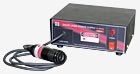Detector with output measurement unit

Model No: ED-P-07-DOMU
Sensor Type
:
Photo Transistor
Display
:
7 segment, 3 ½ digit
Range
:
0 - 199 milli / micro amperes
Quantity
:
1 no.|